Next: B.3 Numerical Evaluation Up: B. Analytical Fourier Transformations Previous: B.1 Triangular Patterns

# B.2 Rectangular Patterns

Similarly a general rectangle {(x1, y1),(x2, y2),(x3, y3),(x4, y4)} in2 can always be transformed to the unit rectangle {(0, 0),(1, 0),(0, 1),(1, 1)} by the transformation (B.2). For rectangular patterns the integral in (B.1) equals to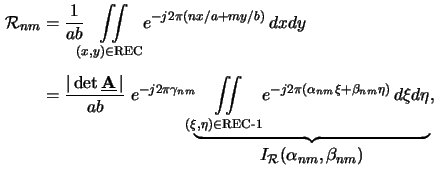whereby REC-1 refers now to the unit rectangle and the coefficients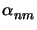,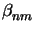and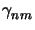are given by (B.5) again. The function I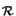(,) is calculated to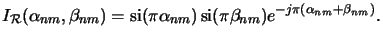(B.6)

Heinrich Kirchauer, Institute for Microelectronics, TU Vienna
1998-04-17